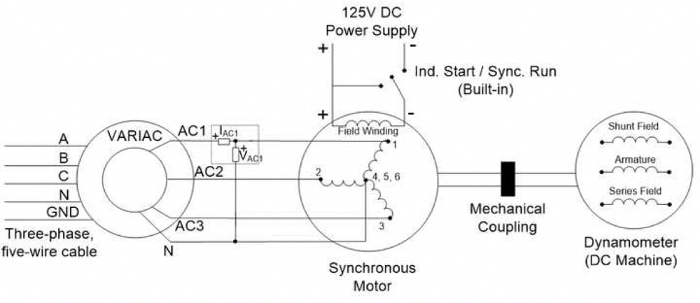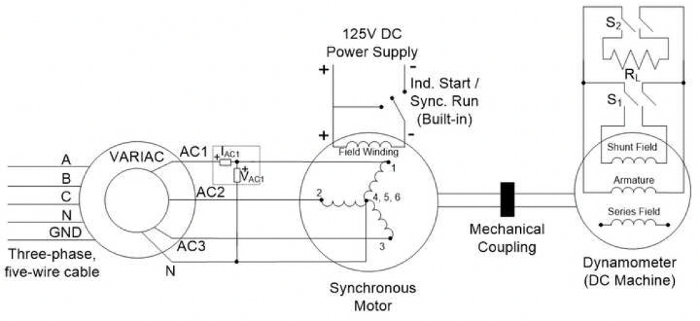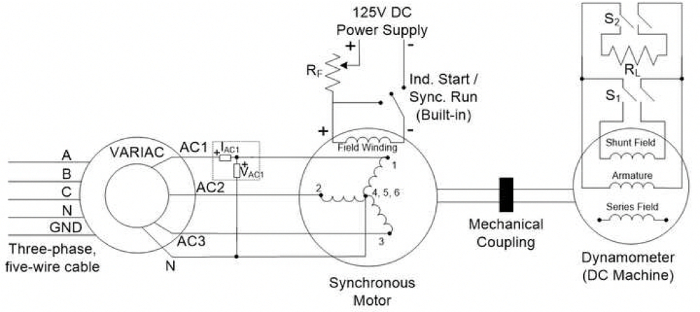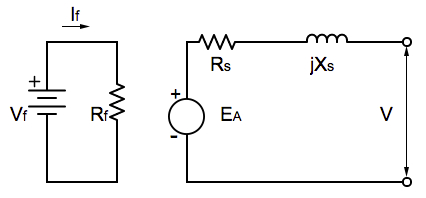Trial ends in
JoVE Science Education
Electrical Engineering

A subscription to JoVE is required to view this content.
You will only be able to see the first 20 seconds.

AC Synchronous Machine Characterization
##### Science Education (Electrical Engineering)
VFD-fed AC Induction Machine
##### Science Education (Electrical Engineering)
AC Induction Motor Characterization

# AC Synchronous Machine Characterization

### Overview

Source: Ali Bazzi, Department of Electrical Engineering, University of Connecticut, Storrs, CT.

Three-phase wound-rotor synchronous motors are less popular than permanent magnet rotor synchronous motors due to the brushes required for the rotor field. Synchronous generators are much more common and available in most existing power plants, as they have excellent frequency and voltage regulation. Synchronous motors have the advantage of almost 0% speed regulation due to the fact that the rotor speed is exactly the same as the stator's magnetic field speed, causing the rotor speed to be constant, irrespective of how much the motor's shaft is loaded. Thus, they are very suitable for fixed speed applications.

The objectives of this experiment are to understand the concepts of starting a three-phase synchronous motor, V-curves for various loads where the load affects the motor power factor, and the effect of loads on the angle between the terminal voltage and back e.m.f.

### Principles

Synchronous machines rely on the rotating magnetic field concept introduced for induction machines. Three-phase currents, flowing in the machine's stator, produce a rotating magnetic field of constant magnitude at a desired frequency. The difference between the synchronous and asynchronous machines is that the latter has shorted windings or a "squirrel cage" on the rotor side, while synchronous machines have a fixed magnetic field on the rotor side. This magnetic field is either provided by an exciter or by permanent magnets. Permanent magnet synchronous machines are becoming more common due to their high efficiency and compact size, but they typically utilize rare earth materials. The term synchronous is used because the rotor magnetic field, which is independent from the stator, locks to the rotating magnetic field and causes the rotor to spin at the same speed (or synchronous speed) as the stator's rotating magnetic field.

To start a three-phase wound-rotor synchronous motor, the field winding is shorted where the machine acts as an induction motor. Once the machine speed is close to synchronous speed, the short circuit is removed and a DC voltage is applied across the field winding. This locks the rotor and stator magnetic fields, and thus, rotor and stator synchronism is achieved. In this lab, the synchronous motor is started by having the top switch on its interface plate in the "Induction Start" position, and once the speed reaches steady state, the switch is flipped to the "Synchronous Run" position.

### Procedure

1. DC Test

1. Turn on the low-power DC power supply with a short circuit across its terminals.
1. Limit the current on the low-power DC power supply to 1.8 A.
2. Turn off the supply and disconnect the short circuit.
2. Connect the supply terminals across ports 1 and 4 of the synchronous motor.
1. Turn on the supply and measure the DC voltage and current. Vary the voltage as needed to reach a current of 1.8 A.
2. Turn off the supply, then repeat the previous two steps for ports 2 and 5 and ports 3 and 6.
3. Disconnect the low-power DC power supply.

2. Synchronous Machine Start-up

1. Make sure the three-phase disconnect switch, synchronous motor switch, and DC motor switch are all off.
1. Check that the VARIAC is at 0%.
2. Wire the VARIAC to the three-phase outlet, and connect the setup shown in Fig. 1.
3. Remember to set the 1 to 1000 scaling of the digital power meter current probe.
2. Check that the "Start/Run" switch is in the "Start" position.
3. Turn on the three-phase disconnect switch.
4. Quickly increase the VARIAC output until the digital power meter reads around 115 V.
5. Measure the armature current IAC1, armature voltage VAC1, real power, and power factor.
6. Remember that the phase (line-to-neutral) voltage and phase current on phase "a" are being measured, so the power factor measurement on the power meter is correctly reflecting the per-phase power factor.
7. Measure the torque and speed of the machine.
8. Turn on the 125 V DC power supply. Make sure all connections are clear from the supply terminals.
1. Press the supply "Start" button and set the supply output to 125 V.
9. Flip the "Start/Run" switch to the "Run" position. Pay attention to how the machine sound changes. The machine sound becomes smoother as the rotor magnetic field locks to the stator rotating magnetic field.
1. Record the armature current IAC1, armature voltage VAC1, real power, power factor, and the field voltage and current from the DC power supply display.
2. Measure and record the torque and speed of the machine.
10. Turn off the DC power supply, flip the "Start/Run" switch to the "Start" position, and set the VARIAC back to 0%.
11. Turn off the three-phase disconnect switch. Leave the rest of the circuit intact.Figure 1: A schematic of the setup to start the synchronous motor. Please click here to view a larger version of this figure.

3. Effect of Load on Torque Angle

1. Make sure the three-phase disconnect switch, synchronous motor switch "S1," and DC motor switch "S2" are all off.
1. Note that while "S1"is on the synchronous motor side, it is used to connect/disconnect the "RL" load across the DC machine terminals.
2. Check that the VARIAC is at 0%.
2. Connect the setup shown in Fig. 2 and set "RL" to 200 Ω.
3. Check that the "Start/Run" switch is in the "Start" position.
4. Turn on the three-phase disconnect switch.
5. Quickly increase the VARIAC output until the digital power meter reads around 115 V.
6. Turn on the 125 V DC power supply. Make sure all connections are clear from the supply terminals.
7. Press the supply start button and set the supply output to 125 V.
8. Flip the "Start/Run" switch to the "Run" position.
9. Record the armature current IAC1, armature voltage VAC1, real power, power factor, and field voltage and current from the DC power supply display.
10. Measure and record the torque and speed of the machine.
11. Keep the strobe light on near the shaft and measure the initial angle δo.
1. To set up the strobe light, plug it into a regular power outlet and turn it on.
2. With the Coarse knob, adjust the speed reading on the strobe light to be close enough to 1,800 RPM, which is the synchronous speed of 1 four-pole 60 Hz machine. Synchronous speed is calculated in rounds per minute (RPM) as n=120xf/P where f is the frequency and P is the number of poles.
3. Place the strobe light to face the motor shaft's edge, and adjust the Fine knob until the shaft appears stationary. The human eye is tricked to see the shaft as stationary by having the strobe light frequency (or speed reading) match the shaft speed.
12. Turn on "S1" and repeat steps 3.9 to 3.11, but measure the new angle as δ1.
13. Turn on "S2" and repeat steps 3.9 to 3.11, but measure the new angle as δ2.
14. Turn off "S2" and change "RL" to 100 Ω.
15. Turn on "S2" and repeat steps 3.9 to 3.11, but measure the new angle as δ3.
16. Turn off the DC power supply, flip the "Start/Run" switch to the "Start" position, turn off "S1"and "S2," and set the VARIAC back to 0%.
17. Turn off the three-phase disconnect switch. Leave the rest of the circuit intact.Figure 2: A schematic of the setup to study the effect of load on torque angle. Please click here to view a larger version of this figure.

4. Effect of Field Current on Power Factor

This section investigates one side of the V-Curve.

1. Make sure the three-phase disconnect switch, synchronous motor switch S1, and DC motor switch S2 are all off.
2. Check that the VARIAC is at 0%.
3. Connect the setup shown in Fig. 3, which is only different from Fig. 2 by adding the series field resistor "RF," and set "RL" to 200 Ω.
4. Set "RF" to the 10 Ω position. "RF" does not require measurement for this experiment, since the goal is to vary field current only.
5. Check that the "Start/Run" switch is in the "Start" position.
6. Turn on the three-phase disconnect switch.
7. Quickly increase the VARIAC output until the digital power meter reads 115 V.
8. Turn on the 125 V DC power supply. Make sure all connections are clear from the supply terminals.
9. Press the supply start button and set the supply output to 125 V.
10. Flip the "Start/Run" switch to the "Run" position.
11. For "RF" = 10, 6, 3, and 1, record the armature current IAC1, armature voltage VAC1, real power, power factor, field voltage and current from the DC power supply display.
12. Measure and record the torque and speed of the machine.
13. Reset "RF" to 10 Ω.
14. Turn on "S1," and repeat steps 4.11 to 4.13.
15. Turn on "S2," and repeat steps 4.11 to 4.13.
16. Turn off "S2" and change "RL" to 100 Ω.
17. Turn on "S2," and repeat steps 4.11 to 4.13.
18. Turn off the DC power supply, flip the "Start/Run" switch to the "Start" position, and set the VARIAC back to 0%.
19. Turn off the three-phase disconnect switch and disconnect the setup.Figure 3: A schematic of the setup to study the effect of changing the field current. Please click here to view a larger version of this figure.

Synchronous motors are ideal in applications requiring constant rotor speed independent of varying shaft load, and are almost ubiquitous in power plants for regulating frequency and voltage. Synchronous machines consist of an inner rotating core called the rotor and an outer stationary ring called the stator. The rotor magnetic field is fixed and is generated using either permanent magnets or a DC power source. In three phase synchronous motors, current flows to the machine's stator with each phase connected to its own separate set of stator coils. This produces a separate rotating magnetic field that corresponds to oscillations in the supply line current. The stator and rotor magnetic fields are coupled or locked, causing the rotor to spin at a speed exactly the same as the stator magnetic field's rotation rate. The overall goal of this video is to introduce three phase synchronous machines, demonstrate protocols for starting and locking the rotor and stator magnetic fields, and illustrate a protocol for finding the effect of motor loads on torque angle.

To overcome initial inertia before the stator and rotor fields are aligned, a three phase synchronous machine is initially run as an induction motor. In this procedure, the stator's rotating magnetic field induces current in the squirrel cage rotor, subsequently creating a magnetic field around the rotor and inducing rotation. Once the machine speed approaches synchronous speed, a DC voltage is applied across the field winding. From this point on, electromagnetic excitation controls the rotor magnetic field. With the rotor magnetic field fixed, the rotor and stator magnetic fields become locked to achieve rotor-stator synchronism. Consequently, the synchronous motor's speed is controlled by the stator magnetic field rotation speed, and is independent of load. While rotor load does not affect the rotor's speed in a synchronous motor, it does cause the rotor pulls to fall slightly behind the stator pulls. While the motor continues to run at synchronous speed, the angular displacement is called the torque angle, which is smaller at lower loads and larger at higher loads. As the mechanical load increases, torque angle increases until the angle is so high that the rotor is pulled out of synchronism. This high mechanical load is therefore above the limit to which the motor can handle, and is called the breakdown torque. Now that synchronous motors have been introduced, we will demonstrate procedures for startup, synchronization, and characterization.

Before starting the synchronous motor, test the DC power supply used to lock the rotor and stator magnetic fields. First, short-circuit the low-powered DC power supply and turn it on. Reduce the current on the supply to one point eight amps, and then turn off the supply and disconnect the short-circuit. The DC test measures the stator winding resistance. First, connect the DC supply terminals across ports one and four of the synchronous motor, and turn on the supply. Then, record the DC voltage and current across these ports. Vary the voltage as needed to reach a current limit of one point eight amps. Record the voltage and then turn off the supply. Repeat the voltage and current measurements as described across ports two and five, and then for ports three and six. Finally, disconnect the DC supply to complete the DC test.

In the next step of the protocol, the synchronous machine is started in induction motor mode, and then the rotor and stator magnetic fields are locked. First, check that the three phase disconnect switch, synchronous motor switch, and DC motor switch are all off. Then, check that the variac is set to zero percent output voltage. With the equipment shut off, wire the variac to the three phase outlet, and connect the setup as shown. Then, attach a small piece of tape to the AC synchronous machine rotor shaft. Finally, set the five to 100 A-scaling of the digital power meter current probe. Now, start the motor by powering the equipment on. First, check that the 'Start-Run' switch is in the 'Start' position. Second, turn on the three phase disconnect switch. Third, quickly increase the variac output until the digital power meter reads around 115 volts. These measurements correspond to phase A, the line to neutral phase voltage and current so that the power factor measurement correctly reflects the per phase power factor. Then, measure the motor torque in induction mode. Finally, measure motor speed using the strobe light technique. Please refer to the Science Education video, "DC Motors" for more information on this technique. With the machine started, and initial parameters measured, it is ready for synchronization. First, turn on the 125 volt DC power supply. Then, flip the 'Start-Run' switch to the 'Run' position. Pay attention to how the machine sound changes. As the rotor magnetic field locks to the stator rotating magnetic field, the machine sound becomes smoother. With rotor and stator magnetic fields locked, or synchronized, measure the armature current and voltage, power, and power factor. Then, measure the field voltage and current from the DC power supply display. Next, measure the mechanical characteristics, torque, and speed. Finally, turn off the equipment starting with the DC power supply. Then, flip the 'Start-Run' switch to the 'Start' position, and set the variac back to zero percent output. Last, turn off the three phase disconnect switch.

When a DC motor is mechanically coupled to the synchronous machine to provide a mechanical load, the torque angle in the synchronous motor can be modified by the shunt field current in the DC motor. This protocol examines the relationship between motor field load and torque angle. With the equipment shut off, connect the set up as shown, and set the shunt load resistor to two kilo ohms. Now, power up the equipment as described previously. Record the electrical and mechanical parameters as before. Next, record torque angle with the shunt field loaded. To do so, use the strobe to visually freeze the shaft of the synchronous motor. Adjust the strobe frequency using the 'course' nob to approximately match 1800 RPM, the synchronous speed of four pull 60 hertz machine. Then, aim the strobe light at the motor shaft's edge, and adjust the 'fine' nob until the shaft appears stationary Initially, measure the torque angle with RL set to 200 ohm, and switches S1 and S2 off. Then, repeat the angle measurements with the shunt field loaded as follows. Flip S1 on and measure angle delta one, then turn S2 on and measure angle delta two. Last, turn off S2, change RL to 300 ohm, and turn S2 back on. Finally, turn off the equipment as described previously.

The DC phase resistance was estimated from the DC test as the ratio of DC voltage to DC current when applied between a phase terminal and the neutral. Phase resistance contributes to losses in the machine, and causes voltage drop across the armature. The field resistance was measured in a similar manner by applying DC voltage to the field winding and measuring the field current. Field resistance controls field current. Field voltage can be varied with a fixed field resistance to vary field current. Finally, the torque angle became larger with increased mechanical load modified by varying the shunt field current in the DC motor. The real power of the machine is then related to the torque angle as shown. This tells us that the output power is highest when the torque angle is zero.

Synchronous machines are common in applications requiring constant speed on the motor's shaft with very tight speed regulations. Three phase wound rotor synchronous generators are the main source of electrical power worldwide. In order to connect the generator at one plant to the electrical grid, three factors in the generator output voltages must match those of the grid, magnitude, frequency, and phase sequence. While automatic synchronizers are usually utilized in large power plants, a simple method is demonstrated for manual synchronization in the Science Education video, "AC Synchronous Machine Synchronization." Synchronous motors are often used for simple devices such as ball mills. A ball mill is a device that blends and grinds materials by rotating a cylinder containing small metal balls. The impact of the balls grinds the materials placed within the cylinder. These grinders are used frequently to blend materials such as paints, or to pulverize materials such as plant grain.

You've just watched Jove's introduction to AC synchronous machine characterization. You should now understand how AC synchronous machines work, how to start and synchronize the machine, and recognize the effect of motor loads on torque angle. Thanks for watching.

### Results

The DC phase resistance can be estimated from the DC test as the ratio of DC voltage to DC current when applied between a phase terminal and the neutral. The field resistance can be measured in a similar manner by applying DC voltage to the field winding and measuring the field current. The synchronous reactance (Xs), back e.m.f. of the machine (EA), and its related constant kφ can be found from the real power (P3φ) measurement into the machine: P3φ=3VφEAcos(δ)/Xs (ignoring the stator resistance Rs) and basic power flow equations for the per-phase equivalent circuit (Fig. 4).

V-curves determine the power factor of the machine as seen by the source (grid). The V-curves demonstrate that the machine can provide reactive power (leading power factor) under certain conditions, and therefore, acts like a capacitor that can enhance voltage stability on the grid. When operating under such a condition, the machine is termed "synchronous condenser."Figure 4: A schematic of the per-phase equivalent circuit used for the representative results.X